Select Page

In the previous article, we started exploring some of the basic machine learning algorithms. There we covered Linear Regression, it’s variations and we implemented it from scratch with Python. We also used other technologies like TensorFlow and Pytorch for the implementation of this algorithm with gradient descent. In this article, we focus on the classification algorithm or to be more precise, the algorithms that are used primarily for classification problems. Note that we will not cover all the classification algorithms, for example, SVM and Decisions Trees, because these algorithms can be used for regression as well, so they will get separated articles just for them.

Are you afraid that AI might take your job? Make sure you are the one who is building it.

STAY RELEVANT IN THE RISING AI INDUSTRY! 🖖

When we are solving classification problems we want to predict the class label of the observed sample. For example, we want to predict a class of penguins based on their bill length and width. There are several approaches to solving this. As we will see some of the solutions are based on calculating distances, while others are based on creating a probabilistic model. One way or another, the goal is to create function y = f(X) that minimizes the error of misclassification, where X is the set of observations and y is the output class label. Before we dive into details of each algorithm let’s check out what we need to install and import.

## Dataset and Prerequisites

Data that we use in this article is from PalmerPenguins Dataset. This dataset has been recently introduced as an alternative to the famous Iris dataset. It is created by Dr. Kristen Gorman and the Palmer Station, Antarctica LTER. You can obtain this dataset here, or via Kaggle. This dataset is essentially composed of two datasets, each containing data of 344 penguins. Just like in Iris dataset there are 3 different species of penguins coming from 3 islands in the Palmer Archipelago. Also, these datasets contain culmen dimensions for each species. The culmen is the upper ridge of a bird’s bill. In the simplified penguin’s data, culmen length and depth are renamed as variables culmen_length_mm and culmen_depth_mm.For the purpose of this article, make sure that you have installed the following Python libraries:

• NumPy – Follow this guide if you need help with installation.
• SciKit Learn – Follow this guide if you need help with installation.

Once installed make sure that you have imported all the necessary modules that are used in this tutorial.

``````import pandas as pd
import numpy as np
import matplotlib.pyplot as plt
from scipy.stats import norm

from sklearn.model_selection import train_test_split
from sklearn.metrics import accuracy_score
from sklearn.preprocessing import StandardScaler
from sklearn.linear_model import LogisticRegression
from sklearn.neighbors import KNeighborsClassifier
from sklearn.naive_bayes import GaussianNB``````

Apart from that, it would be good to be at least familiar with the basics of linear algebra, calculus and probability.

## Logistic Regression

The first algorithm that we explore in this article is Logistic Regression. The name might be a bit confusing because it comes from statistics and it is due to the similar mathematical formulation for Linear Regression. Just so simplify things even more for this first algorithm, we explain it in the case of binary classification, meaning we have only two classes. As we mentioned, this algorithm has a similar formulation as linear regression. What we want to do is to model yi as a linear function of xi, but that is not as simple now when yi can have only two values (two classes remember). So, the Logistic Regression model still computes a weighted sum of the input features and adds a bias term, but instead of outputting the result directly, it does some extra processing.

That is why we assign value 0 to the first class (negative class) and value 1 to the second class (positive class). That is how the problem is transformed into the problem of finding a continuous function whose codomain is (0, 1). This means that we want to estimate the probability that an observed sample belongs to a particular class. For that purpose, sigmoid function or standard logistic function is used:So, with Logistic Regression we still calculate wX + b (or to simplify it even further and put all parameters into the matrix – θX) value and put the result in sigmoid function. If the result is greater than 0.5 (probability is larger than 50%), then the model predicts that the instance belongs to that class positive class(1), or else it predicts that it does not belong to it (negative class). Mathematically we can put it like this: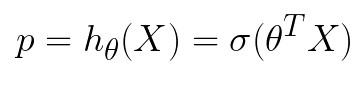It is important to note that we need to modify loss function as well in order for it to work on this type of data. For this purpose we use log loss function which is defined like this: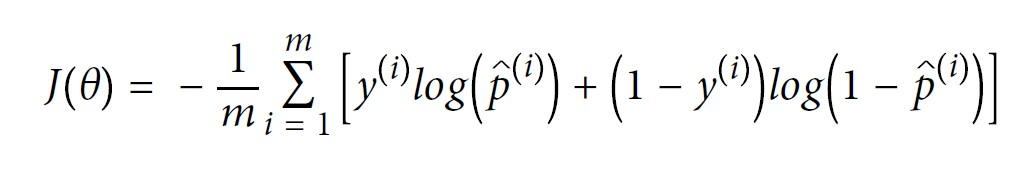Unlike the loss function that we used for the Linear Regression, this formula doesn’t have its closed form. We can not use the Normal Equation, so we need to use gradient descent to optimize it. For that purpose we need to calculate partial derivatives of the cost function with regards to the jth model parameter θj: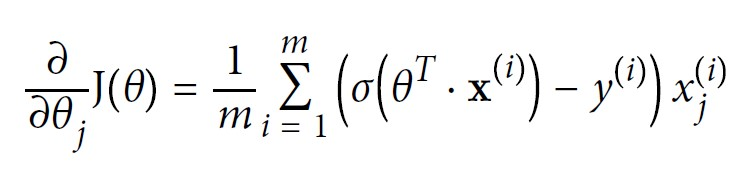Ok, that would be rough theory behind it, let’s move to the implementation.

### Preparing data for Logistic Regression

Let’s first load and prepare data for Logistic Regression. We perform binary classification, so we don’t use all data from the PalmerPenguins dataset. Here is the complete data when we load it:

``````data = pd.read_csv('./data/penguins_size.csv')We remove all samples that are labeled with class ‘Chinstrap‘ and features that we don’t want to use (we use only culmen_length_mm and culmen_depth_mm).

``````data = data.dropna()
data = data.drop(['sex', 'island', 'flipper_length_mm', 'body_mass_g'], axis=1)
data = data[data['species'] != 'Chinstrap']``````

Ok, now data is ready so we can define input data, output data and split it into train and test sets.

``````X = data.drop(['species'], axis=1)
X = X.values
ss = StandardScaler()
X = ss.fit_transform(X)

y = data['species']
spicies = {'Adelie': 0, 'Gentoo': 1}
y = [spicies[item] for item in y]
y = np.array(y)

# Split the data
X_train, X_test, y_train, y_test = train_test_split(X, y, test_size=0.2, random_state=33)``````

Note that we scale input data using StandardScaler. Just for more transparency here is how to input data and output data looks like when we plot it:

``````plt.figure(figsize=(11, 5))
plt.scatter(X[y == 0][:, 0], X[y == 0][:, 1], color='orange', label='Adelie')
plt.scatter(X[y == 1][:, 0], X[y == 1][:, 1], color='gray', label='Gentoo')
plt.legend();``````### Python Implementation

Let’s implement a class for Logistic Regression from scratch using Python and NumPy. The implementation is in the class MyLogisticRegression:

``````class MyLogisticRegression():
'''Implements algorithm for Logistic Regression'''
def __init__(self, learning_rate=0.1):
self.learning_rate=learning_rate

def __sigmoid(self, x):
return 1 / (1 + np.exp(-x))

def __loss(self, p, y):
return (-y * np.log(p) - (1 - y) * np.log(1 - p)).mean()

def __extend_input(self, X):
ones = np.ones((X.shape, 1))
return np.concatenate((ones, X), axis=1)

return np.dot(X.T, (p - y)) / y.size

def fit(self, X, y, epochs):
X = self.__extend_input(X)

self.theta = np.zeros(X.shape)

for i in range(epochs):
z = np.dot(X, self.theta)
h = self.__sigmoid(z)

loss = self.__loss(h, y)

if(i % 10000 == 0):
print(f'Epoch {i} - Loss: {loss} \t')

self.theta -= self.learning_rate * self.__gradient(X, h, y)

def predict(self, X):
X = self.__extend_input(X)
probability = self.__sigmoid(np.dot(X, self.theta))

return probability.round()``````

Since we use gradient descent for optimization, we need to initialize the learning rate through the constructor. Apart from that, there are four internal functions:

• __sigmoid – Performs sigmoid function on the input information.
• __loss – Calculates log loss
• __gradient – Calculates gradient based on the input data, estimated values and actual class label
• __extend_input – Just like for Linear regression we need to extend input data with ones so we can add it automatically as the bias term.The two public methods fit and predict are used to train the model and to create new predictions for new samples. In the fit method, we first extend the input and initialize theta – the set of parameters. Then we calculate the theta based on the input data and use them along with the sigmoid function to calculate the predictions. After that, we can calculate the loss. Finally, we calculate the gradient and use the learning rate to adjust theta, ie. we perform gradient descent. The predict method just extends input data and gets the probability based on the current parameters.

Finally, let’s use the class on prepared data:

``````model = MyLogisticRegression()
model.fit(X_train, y_train, 200000)

predictions = model.predict(X_test)
predict(accuracy_score(predictions, y_test))``````
``1.0``

Simple, we first create an object of the MyLogisticRegression class, then we call the fit method with the training data and call the predict method on the test data. We call the SciKit Learn accuracy score, which just gets a percentage of the times prediction aligns with the real value. We had a 100% score, meaning we correctly predicted all values in the test set. Let’s visualize that:

``````plt.figure(figsize=(11, 5))
plt.scatter(X[y == 0][:, 0], X[y == 0][:, 1], color='orange', label='Adelie')
plt.scatter(X[y == 1][:, 0], X[y == 1][:, 1], color='gray', label='Gentoo')
plt.legend()

x_values = [np.min(X[:, 0]), np.max(X[:, 1])]
y_values = - (model.theta + np.dot(model.theta, x_values)) / model.theta

plt.plot(x_values, y_values, color='black');``````We can even see our linear model presented as a line which separates two classes. This is exatcly what we wanted.

### Using SciKit Learn

Of course, we don’t need to implement this algorithm on our own. Logistic regression is available as a module in SciKit Learn library. Here is how you can use it:

``````model = LogisticRegression(C=1e20)
model.fit(X_train, y_train)

preditions = model.predict(X_test)
print(accuracy_score(preditions, y_test))``````
``1.0``

Pretty much like our from-scratch implementation. The accuracy is again 100%. The principle we used here can be extended to more classes and features.

## K-Nearest Neighbours (KNN)

Unlike Logistic Regression, this algorithm is not calculating probabilities but is based on distances. This effectively means that it is non-parametric models, but it also means that it keeps all training data in memory after the training. In fact, storing training data is the training process. Basically, once a new previously unseen sample is passed into the algorithm it calculates k training examples that are closest to x and returns the majority label (or average label, depending on the implementation). The distances can be calculated in various different ways. Euclidean distance or Cosine similarity are often used in practice, but you can play around with Manhattan distance or Chebychev distance. In this article we use Euclidean distance which can be described with the formula:To sum it up, this algorithm is simple and intuitive and it can be broken down into several steps:

• Decide the number of neighbors that algorithm considers
• Store training data with corresponding labels in memory
• Once a new input point comes in, calculate it’s the distance from the training points based on the distance function of your choosing
• Sort the results and pick k points that are closest to the new input sample
• Detect the label of the majority of k points and assign this label to new input sampleLet’s implement it in Python.

### Preparing Data for KNN

For this algorithm, we don’t consider binary classification, but the full classification problem with three classes from PalmerPenguins dataset. Effectively this only means that we don’t remove Chinstrap class from the dataset, the other preprocessing steps remain the same.

``````data = pd.read_csv('./data/penguins_size.csv')
ss = StandardScaler()

data = data.dropna()
data = data.drop(['sex', 'island', 'flipper_length_mm', 'body_mass_g'], axis=1)

# Prepare input
X = data.drop(['species'], axis=1)
columns = X.columns
X = X.values
X = ss.fit_transform(X)

# Prepare target
y = data['species']
spicies = {'Adelie': 0, 'Chinstrap': 1, 'Gentoo': 2}
y = [spicies[item] for item in y]
y = np.array(y)

# Split the data
X_train, X_test, y_train, y_test = train_test_split(X, y, test_size=0.2, random_state=33)``````

Here is how that looks like when we plot it:

``````plt.figure(figsize=(11, 5))
plt.scatter(X[y == 0][:, 0], X[y == 0][:, 1], color='orange', label='Adelie')
plt.scatter(X[y == 1][:, 0], X[y == 1][:, 1], color='black', label='Chinstrap')
plt.scatter(X[y == 2][:, 0], X[y == 2][:, 1], color='gray', label='Gentoo')
plt.legend();``````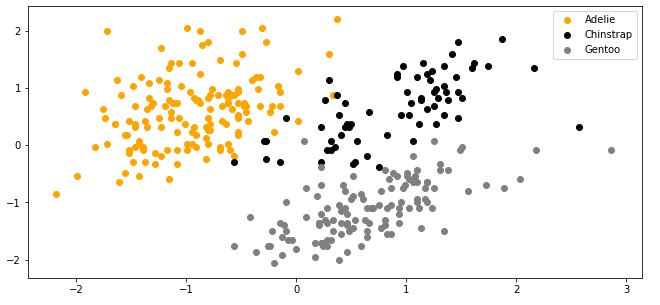### Python Implementation

The whole algorithm is implemented within MyKNearestNeighbors class:

``````class MyKNearestNeighbors():
'''Implements algorithm for K-Nearest Neighbors'''
def __init__(self, num_neighbors=5, num_clasess=3):
self.num_neighbors = num_neighbors
self.num_clasess = num_clasess

def __euclidian_distance(self, a, b):
return np.sqrt(np.sum((a - b)**2, axis=1))

def __get_neighbours(self, X_test, return_distance=False):
neighbours = []

test_train_distances = [self.__euclidian_distance(x_test, self.X_train) \
for x_test in X_test]

for row in test_train_distances:
enumerated_neighbours = enumerate(row)
sorted_neighbours = sorted(enumerated_neighbours, key=lambda x: x) \
[:self.num_neighbors]

index_list = [tup for tup in sorted_neighbours]

neighbours.append(index_list)

return np.array(neighbours)

def fit(self, X_train, y_train):
self.X_train = X_train
self.y_train = y_train

def predict(self, X_test):
neighbors = self.__get_neighbours(X_test)
preditions = np.array([
np.argmax(np.bincount(self.y_train[neighbor]))
for neighbor in neighbors
])

return preditions``````

There are two private methods:
__euclidian_distance – Calculates Euclidean distance between two samples in the dataset
__get_neighbours – Calculates the distance between all points in the train set and input set and returns k neighbors for each sample from the input set.

The two public methods fit and predict are used as an interface to this class. The first one just stores training data in the class attribute. The predict method puts it all together. Basically, it calls __get_neighbours method and utilizes that result to assign labels to every sample of the input set. Usage is the same as for previous algorithms:

``````model = MyKNearestNeighbors()
model.fit(X_train, y_train)

preditions = model.predict(X_test)
print(accuracy_score(preditions, y_test))``````

The accuracy score is once again 100%. We can confirm it by creating pandas data frame with both actual values and predictions:

``````pd.DataFrame({
'Actual Value': y_test,
'KNN Predictions': preditions,
})``````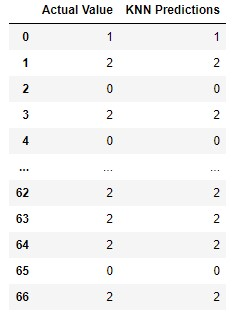### Using SciKit Learn

SciKit Learn of course, provides a class for KNN called KNeighborsClassifier:

``````model = KNeighborsClassifier(n_neighbors=5)
model.fit(X_train, y_train)

print(model.score(X_test, y_test))

sk_knn_preditions = model.predict(X_test)

pd.DataFrame({
'Actual Value': y_test,
'KNN Predictions': preditions,
'SciKit Learn KNN': sk_knn_preditions,
})``````
``1.0``The accuracy using this approach is also 100%. This way we have the same results as with our implementation, which is quite cool.

## Naive Bayes

Third and final algorithm we explore today is the Naive Bayes algorithm. As we mentioned previously, classification problems can be solved by creating a predictive model. That is what we have done with Logistic Regression. Another way to create a predictive model would be to estimate the conditional probability of the class label, given the observation. Meaning, we can calculate conditional probability for each class label in and the pick the label with the highest probability as most likley label. In theory, Bayes Theorem can be used for this: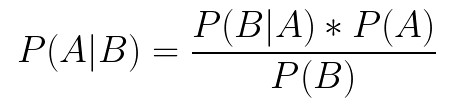The main problem with this approach is that we need a really large dataset to calculate the conditional probability P(x1, x2, …, xn | yi), because this formula assumes that each input variable is dependent upon all other variables. If the number of features is large, the size of the dataset becomes an even bigger problem. To simplify this problem we assume that each input variable as being independent of each other. This might sound weird…because it is 🙂 In reality, it is really rare that input features don’t depend on each other. However, this approach proved to surprisingly well in the wild. That is why we can rewrite the formula from above as:To calculate P(yi) all we have to do is divide the frequency of class yi in the training dataset and divide it with the total number of samples in the training set (P(yi) = # of samples with yi / total # of examples). The second part of the equation, the conditional probability, can be derived from data as well. So, let’s implement it.

### Preparing Data for Naive Bayes

Before we dive into the algorithm implementation let’s prepare the PalmerPenguins dataset again. It is very simmilar for the preparation we have done for KNN:

``````data = pd.read_csv('./data/penguins_size.csv')
ss = StandardScaler()

data = data.dropna()
data = data.drop(['sex', 'island', 'flipper_length_mm', 'body_mass_g'], axis=1)

# Prepare input
X = data.drop(['species'], axis=1)
columns = X.columns
X = X.values
X = ss.fit_transform(X)

# Prepare target
y = data['species']
spicies = {'Adelie': 0, 'Chinstrap': 1, 'Gentoo': 2}
y = [spicies[item] for item in y]
y = np.array(y)

# Split the data
X_train, X_test, y_train, y_test = train_test_split(X, y, test_size=0.2, random_state=33)``````

The only difference is that we stored columns in the columns variable because we need it for the algorithm implementation.

### Python Implementation

The implementation of this algorithm is simple and can be found in the class MyNaiveBias. Note that we utilized the Pandas library a bit more because it provides easy solutions for grouping data:

``````class MyNaiveBias():
'''Implements algorithm for Naive Bias'''
def __init__(self, input_columns):
self.input_columns = input_columns

def fit(self, X_train, y_train):
X_train = pd.DataFrame(X_train, columns = self.input_columns)

self.classes = np.unique(y_train)
self.means = X_train.groupby(y_train).apply(np.mean)
self.stds = X_train.groupby(y_train).apply(np.std)
self.probabilities = X_train.groupby(y_train).apply(lambda x: len(x)) / X_train.shape

def predict(self, X_test):
X_test = pd.DataFrame(X_test, columns = self.input_columns)
predictions = []

for i in range(X_test.shape):
p = {}

for c in self.classes:
p[c] = self.probabilities[c]

for index, row in enumerate(X_test.iloc[i]):
p[c] *= norm.pdf(row, self.means.iloc[c, index], self.stds.iloc[c, index])

predictions.append(pd.Series(p).values.argmax())

return predictions``````

So, the fit method calculating statistics for the training dataset. We store all classes, calculate means and standard deviations for input training data grouped by each class. Finally, we calculate prior probability for each class. The predict method is doing all the hard work here and utilizes those calculations. First, for each sample from the input set and for each class we calculate conditional probability and multiply it with the prior probability for that class. In the end, for each input sample, we pick the class with the largest probability. We use this class as the ones before:

``````model = MyNaiveBias(columns)

model.fit(X_train, y_train)
predictions = model.predict(X_test)

print(accuracy_score(preditions, y_test))

pd.DataFrame({
'Actual Value': y_test,
'Naive Bias Predictions': preditions,
})``````

### Using SciKit Learn

We can use SciKit Learn implementation of Naive Bayes like this:

``````model = GaussianNB()
model.fit(X_train, y_train)
sk_nb_preditions = model.predict(X_test)

print(accuracy_score(sk_nb_preditions, y_test))

pd.DataFrame({
'Actual Value': y_test,
'Naive Bias Predictions': preditions,
'SciKit Learn NB': sk_nb_preditions,
})``````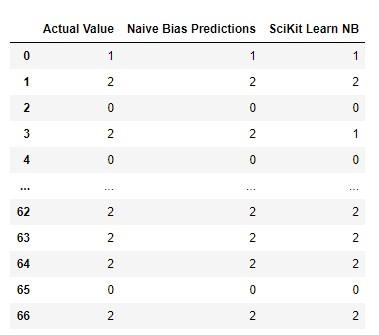## Conclusion

In this article, we covered three classification algorithms that are often used. We explored Logistic Regression, KNN and Naive Bayes. We had a chance to see how they function under the hood and implement them with Python. Also, we had a chance to use out-of-the-box solutions form SciKit Learn. They are simple, but they are a great starting point before going to more advanced algorithms.#### Nikola M. Zivkovic

CAIO at Rubik's Code

Nikola M. Zivkovic a CAIO at Rubik’s Code and the author of book “Deep Learning for Programmers“. He is loves knowledge sharing, and he is experienced speaker. You can find him speaking at meetups, conferences and as a guest lecturer at the University of Novi Sad.

Rubik’s Code is a boutique data science and software service company with more than 10 years of experience in Machine Learning, Artificial Intelligence & Software development. Check out the services we provide.

Ultimate Guide to Machine Learning with Python

Everything from Python basics to the deployment of Machine Learning algorithms to production in one place.

Become a Machine Learning Superhero TODAY!